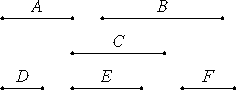# Proposition 26

Similar plane numbers have to one another the ratio which a square number has to a square number.

Let A and B be similar plane numbers.

I say that A has to B the ratio which a square number has to a square number.VIII.18

Since A and B are similar plane numbers, therefore one mean proportional number C falls between A and B.

Take D, E, and F, the least numbers of those which have the same ratio with A, C, and B

VIII.2,Cor

Then the extremes of them D and F are square. And since D is to F as A is to B, and D and F are square, therefore A has to B the ratio which a square number has to a square number.

Therefore, similar plane numbers have to one another the ratio which a square number has to a square number.

Q.E.D.

## Guide

This proposition is used in propositions IX.10 and X.9.